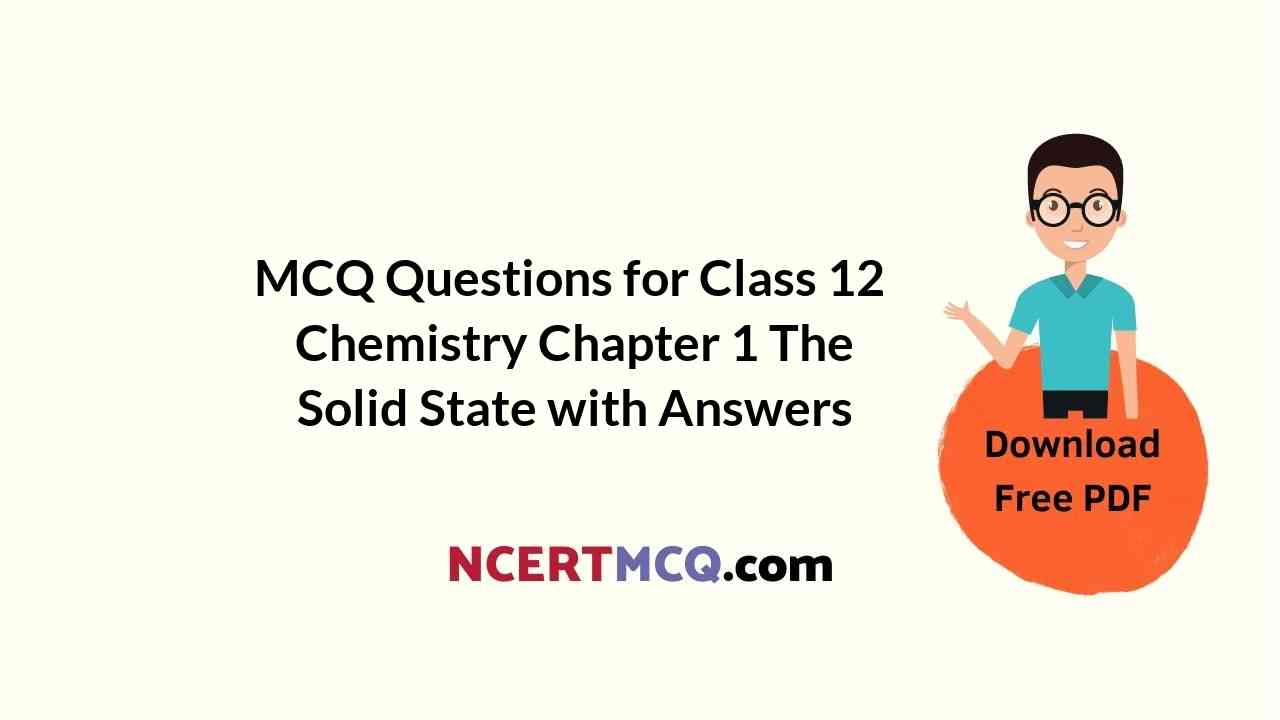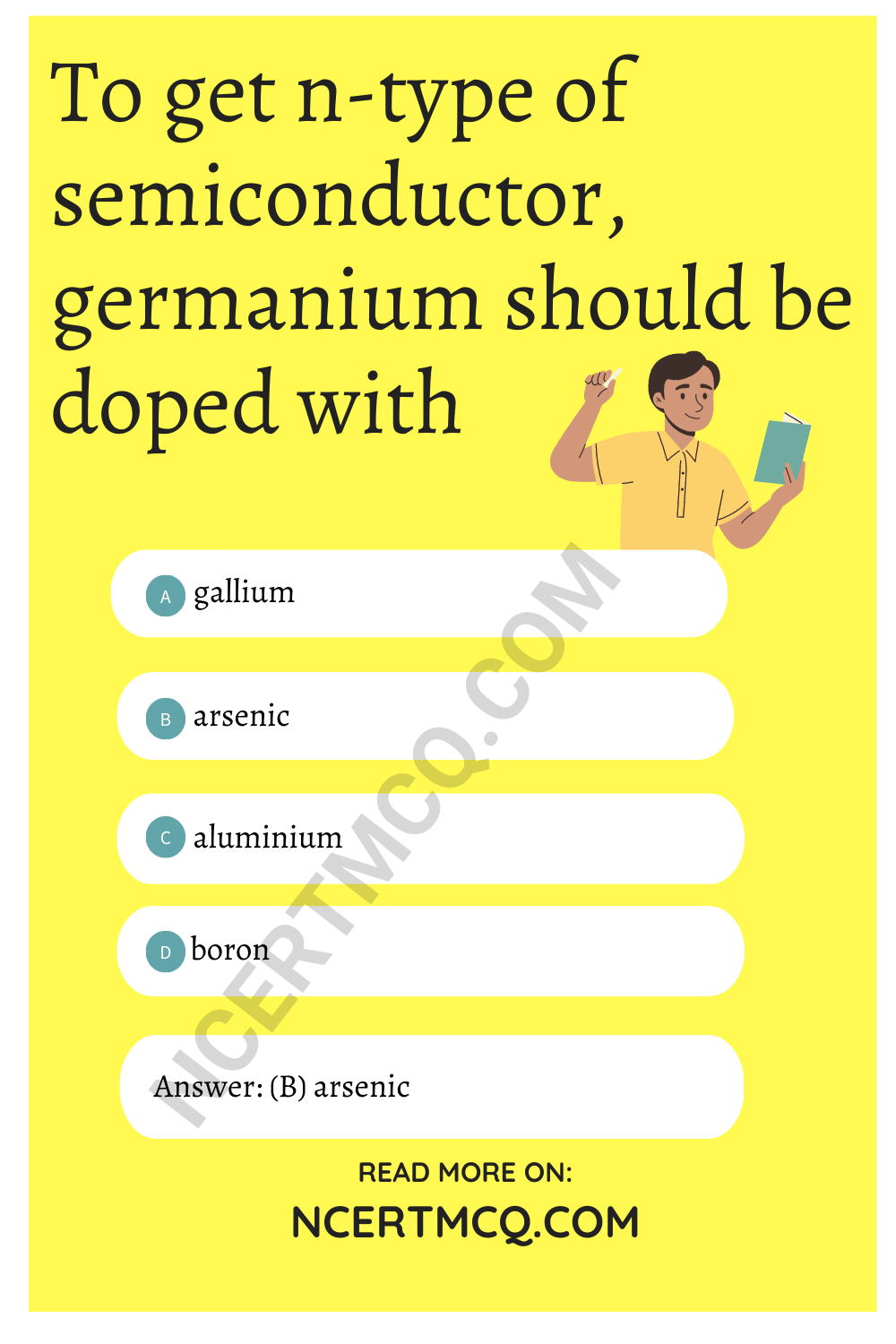Check the below NCERT MCQ Questions for Class 12 Chemistry Chapter 1 The Solid State with Answers Pdf free download. MCQ Questions for Class 12 Chemistry with Answers were prepared based on the latest exam pattern. We have provided The Solid State Class 12 Chemistry MCQs Questions with Answers to help students understand the concept very well.

## The Solid State Class 12 MCQs Questions with Answers

Solid State MCQ Question 1.
Which one of the following is non-crystalline or amorphous?
(a) Diamond
(b) Graphite
(c) Glass
(d) Common Salt

Solid State Class 12 MCQ Question 2.
NaCl typecrystal (with coordination no. 6 : 6) can be converted into CsCl type crystal (with coordination no. 8 : 8) by applying
(a) high temperature
(b) high pressure
(c) high temperature and high pressure
(d) low temperature and low pressure

Solid State MCQs Class 12 Question 3.
How many chloride ions are surrounding sodium ion in sodium chloride crystal ?
(a) 4
(b) 8
(c) 6
(d) 12

Class 12 Chemistry Chapter 1 MCQ Question 4.
In NaCl structure
(a) all octahedral and tetrahedral sites are occupied
(b) only octahedral sites are occupied
(c) only tetrahedral sites are occupied
(d) neither octahedral nor tetrahedral sites are occupied

Answer: (b) only octahedral sites are occupied

Class 12 Chemistry Chapter 1 MCQs Question 5.
In Zinc blende structure
(a) zinc ions occupy half of the tetrahedral sites
(b) each Zn2- ion is surrounded by six sulphide ions
(c) each S2- ion is surrounded by six Zn2+ ions
(d) it has fee structure

Answer: (c) each S2- ion is surrounded by six Zn2+ ions

Solid State MCQ Questions For Class 12 Question 6.
A unit cell of BaCl2 (fluorite structure) is made up of
(a) four Ba2+ ions and four Cl ions
(b) four Ba2- ions and eight Cl ions
(c) eight Ba² ions and four Cl ions
(d) four Ba² ions and six Cl ions

Answer: (b) four Ba2- ions and eight Cl ions

Class 12 Chemistry MCQ Chapter 1 Question 7.
Alkali halids do not show Frenkel defect because
(a) cations and anions have almost equal size
(b) there is a large difference in size of cations and anions
(c) cations and anions have low coordination number
(d) anions cannot be accommodated in voids

Answer: (a) cations and anions have almost equal size

MCQ On Solid State Class 12 Question 8.
The coordination number of Y will be in the XY types of crystal:
(a) 6
(b) 8
(c) 12
(d) 4

Solid State Chemistry Class 12 MCQ Question 9.
The fraction of the total volume occupied by the atoms present in a simple cube is
(a) $$\frac{π}{4}$$
(b) $$\frac{π}{6}$$
(c) $$\frac{π}{3√2}$$
(d) $$\frac{π}{4√2}$$

Answer: (b) $$\frac{π}{6}$$

Chemistry Class 12 Chapter 1 MCQ Question 10.
Edge length of unit cell of chromium metal is 287 pm with bcc arrangement. The atomic radius is of the order
(a) 287 pm
(b) 574 pm
(c) 124.27 pm
(d) 143.5 pmMCQ Solid State Class 12 Question 11.
The edge length of fee cell is 508 pm. If radius of cation is 110 pm, the radius of anion is
(a) 110 pm
(b) 220 pm
(c) 285 pm
(d) 144 pm

Chapter 1 Chemistry Class 12 MCQ Question 12.
The density of a metal which crystallises in bcc lattice with unit cell edge length 300 pm and molar mass 50 g mol-1 will be
(a) 10 g cm-3
(b) 14.2 g cm-3
(c) 6.15 g cm-3
(d) 9.3 2 g cm-3

Class 12 Solid State MCQ Question 13.
How many lithium atoms are present in a unit cell with edge length 3.5 Å and density 0.53 g cm-3? (Atomic mass of Li = 6.94):
(a) 2
(b) 1
(c) 4
(d) 6

MCQ Questions On Solid State With Answers Pdf Question 14.
The distance between Na and CL ions in NaCl with a density 2.165 g cm-3 is
(a) 564 pm
(b) 282 pm
(c) 234 pm
(d) 538 pm

MCQ Of Solid State Class 12 Question 15.
An element with atomic mass 100 has a bcc structure and edge length 400 pm. The density of element is
(a) 10.37 g cm-3
(b) 5.19 g cm-3
(c) 7.29 g cm-3
(d) 2.14 g cm-3

Question 16.
Fe3O4 (magnetite) is an example of
(a) normal spinel structure
(b) inverse spinel structure
(c) fluoride structure
(d) anti fluorite structure

Question 17.
Which of the following crystals does not exhibit Frenkel defect?
(a) AgBr
(b) AgCl
(c) KBr
(d) ZnS

Question 18.
What type of stoichiometric defect is shown by ZnS?
(a) Schottky defect
(b) Frenkel defect
(c) Both Frenkel and Schottky defects
(d) Non-stoichiometric defect

Question 19.
Silver halides generally show
(a) Schottky defect
(b) Frenkel defect
(c) Both Frenkel and Schottky defects
(d) cation excess defect

Answer: (c) Both Frenkel and Schottky defects

Question 20.
Which of the following will have metal deficiency defect?
(a) NaCl
(b) FeO
(c) KCl
(d) ZnO

Question 21.
In which pair most efficient packing is present?
(a) hep and bcc
(b) hep and ccp
(c) bcc and ccp
(d) bcc and simple cubic cell

Question 22.
What is the effect of Frenkel defect on the density of ionic solids?
(a) The density of the crystal increases
(b) The density of the crystal decreases
(c) The density of the crystal remains unchanged
(d) There is no relationship between density of a crystal and defect present in it

Answer: (c) The density of the crystal remains unchanged

Question 23.
In a Schottky defect
(a) an ion moves to interstitial position between the lattice points
(b) electrons are trapped in a lattice site
(c) some lattice sites are vacant
(d) some extra cations are present in interstitial space

Answer: (c) some lattice sites are vacant

Question 24.
To get n-type of semiconductor, germanium should be doped with
(a) gallium
(b) arsenic
(c) aluminium
(d) boronQuestion 25.
p-type semiconductors are formed When Si or Ge are doped with
(a) group 14 elements
(b) group 15 elements
(c) group 13 elements
(d)group 18 elements

Question 26.
Which of the following conditions favours the existence of a substance in the solid state?
(a) High temperature
(b) Low temperature
(c) High thermal energy
(d) Weak cohesive forces